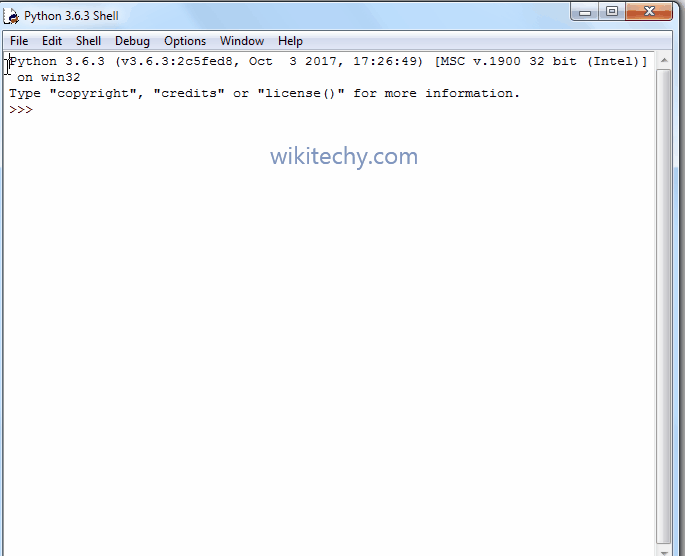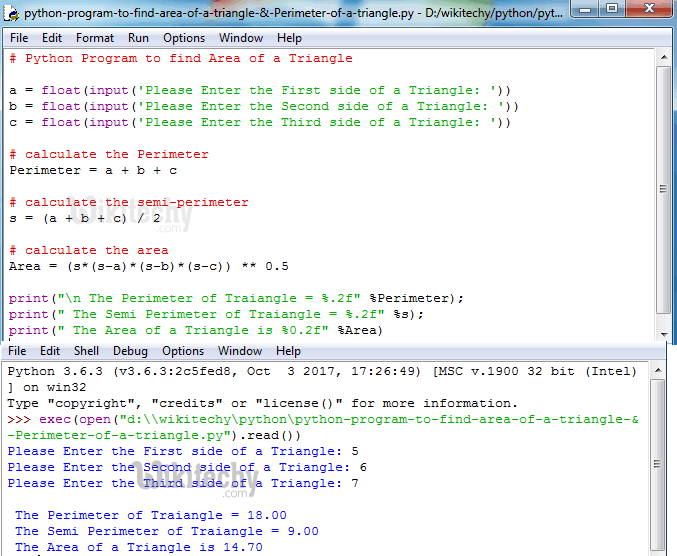# python tutorial - Python Program to find Area Of a Triangle - learn python - python programming

• To write Python Program to find Area Of a Triangle, Perimeter of a Triangle and Semi Perimeter of a Triangle with example.
• Before we step into the program, Let see the definitions and formulas behind Perimeter and Area Of a Triangle.

## Area Of a Triangle

• If we know the length of three sides of a triangle then we can calculate the area of a triangle using Heron’s FormulaLearn Python - Python tutorial - Python Program To find Area Of Circle - Python examples - Python programs

• Where s = (a + b + c )/ 2 (Here s = semi perimeter and a, b, c are the three sides of a triangle)

## Python Program to find Area of a Triangle & Perimeter of a Triangle

• This Python program allows the user to enter three sides of the triangle. Using those values we will calculate the Perimeter of a triangle, Semi Perimeter of a triangle and then Area of a Triangle.

## OutputLearn Python - Python tutorial - Python Program to find Area of a Triangle & Perimeter of a Triangle - Python examples - Python programs

## Analysis

• Following statements will allow the User to enter the three sides of the triangle a, b, c.
• Next, Calculating the Perimeter of Triangle using the formula P = a+b+c.
• Next, Calculating the semi perimeter using the formula (a+b+c)/2.
• Although we can write semi perimeter = (Perimeter/2) but we want show the formula behind. That is why we used standard formula
• Calculating the Area of a triangle using Heron’s Formula:

## Python Program to find Area of a Triangle using functions

• This program allows the user to enter three sides of the triangle.
• We will pass those three values to the function arguments to calculate the area of a triangle.

## OutputLearn Python - Python tutorial - Python Program to find Area of a Triangle using functions - Python examples - Python programs

## Analysis

• First, We imported the math library using the following statement.
• This will allow us to use the mathematical functions like math.sqrt function
• Next, We defined the function with three arguments using def keyword. It means, User will enter the three sides of the triangle a, b, c.
• Calculating the Area of a triangle using Heron’s Formula: sqrt(s*(s-a)*(s-b)*(s-c)); (sqrt() is the mathematical function inside the math library, which is used to calculate the square root.

NOTE: Please be careful while placing the open and close brackets, it may change the entire calculation if you place it wrong